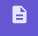# Activity #22__Construct a 30 - 60 - 90 Triangle

## Activity Goal

Construct the 30- 60- 90 triangle (shown below) and then find the missing side lengths.

## DIRECTIONS (if you need help)

1. Use the Segment with Given Size tool to create the line segment AB with a length of 9. 2. Construct A = 30 (notice that the point B' appears; we will need to delete this point later). 3. Construct a Ray AB'. 4. Construct B = 90 (notice that the point A' appears, will delete this point also later) . 5. Construct Ray BA'. 6. Use the Points tool to choose the intersect tool and create the point C (where the two rays intersect). 7. Click Steps Menu,, hide the rays and the points A' and B' (unclick each) . 8. Now get out of the Steps Menu and back into the Tools Menu . 9. Use the Polygon tool to create the triangle ABC -- as shown above. 10. Use the Move tool, to find the lengths of side a and side b.